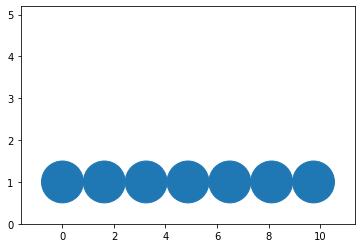# Different outputs in notebook and script

I assume this has to do with misusing the API and `axis.transData`, but wanted to check if there is anything obvious to fix here: My goal is to

• have a bunch of circular markers
• have them just touch at the edges

Here is a stripped down version of the function:

``````import matplotlib.pyplot as plt
import numpy as np

def funny_stuff(axis=None):
np.random.seed(1)
centers = np.arange(0, 10, step=np.abs(np.random.randn()))
if axis is None:
axis = plt.gca()

circs = axis.scatter(centers, np.ones_like(centers))

bin_width = centers - centers
axis.set_xlim(centers - bin_width, centers[-1] + bin_width)

axis.set_ylim(0, 2)

diff = axis.transData.transform([(0, 1), (bin_width, 0), (0, 0)])
x_scale = max(diff - diff[-1])
y_scale = max(diff - diff[-1])

if x_scale > y_scale:
axis.set_ylim(0, (x_scale / y_scale) * 2)
diff = axis.transData.transform([(0, 1), (0, 0)])

size = max(diff - diff[-1])
circs.set_sizes([size ** 2 for _ in centers])
return axis
``````

If I run this in a jupyter notebook, it works as desired:If I instead run this in a script (using either `plt.show` or `savefig`), I get something different (I am a new user, and can include only one image), and the circles are overlapping.

I have tried setting backends, and playing with arguments to `savefig`, but have had no luck. If I call `savefig` from the notebook, it works as desired.

For reasons that are unclear to me `circ.set_sizes` takes a `dpi` argument which is hard-coded to 72. Inline may still default to 72dpi whereas with the other backends we default to 100dpi now. That probably should look at the figure to get the default (and fallback to 72 if the artist is not in a figure with a dpi?)

The units on `set_size` are square points (which does sort of make sense for scatter). If you want a relatively small number of just touching spheres I suggest using `matplotlib.patch.Circle` directly.

Thanks for the help!

The suggested fix did not work, but calling `axis.figure.set_dpi(72.0)` as early as possible does work. I am guessing that somewhere there is some logic in `scatter` that uses this…

For aesthetic reasons, I want things that look like circles, which is why I am not using `Circle`, since it can look like an ellipse depending on the underlying coordinates! The method I used does surprisingly well for how many hacks it is built on.

Hopefully I’ll have something to share tomorrow1 Like